# In-time analysis problem

This post tries to explain the basic concepts in a time series EDA using the in-time analysis problem. I want to analyze my entry time at the office and understand how different factors affect it.

The dependent variable is diff.in.time which is the difference between policy in-time and actual in-time in office. A sample of the data is shown (Actual data is not shown for security reasons. This is mock data which is very similar to the actual one. The analysis will be the same).

Sample Data
Attendance.Date diff.in.time diff.out.time
232 2017-12-06 19 mins 3 mins
186 2018-02-15 37 mins -6 mins
163 2018-03-20 37 mins 232 mins
84 2018-07-18 -11 mins 4 mins
73 2018-08-03 1 mins 48 mins

The first thing to do in any data analysis task is to visualize the data. Graphs help in visualizing various features of the data, including patterns, unusual observations, change over time, and relationships between variables.

## Time plot

For time series data, the basic graph to start with is a time plot. In this plot, the dependent variable is plotted against the time, with consecutive observations joined by straight lines. In this problem, diff.in.time will be plotted against the date of observation.

library(xts)
library(forecast)
time.series <- xts(attendance$diff.in.time, order.by= attendance$Attendance.Date)
autoplot(time.series) +
ggtitle("Difference of actual in-time vs policy in-time") +
xlab("Time") + ylab("Minutes") +
theme_minimal()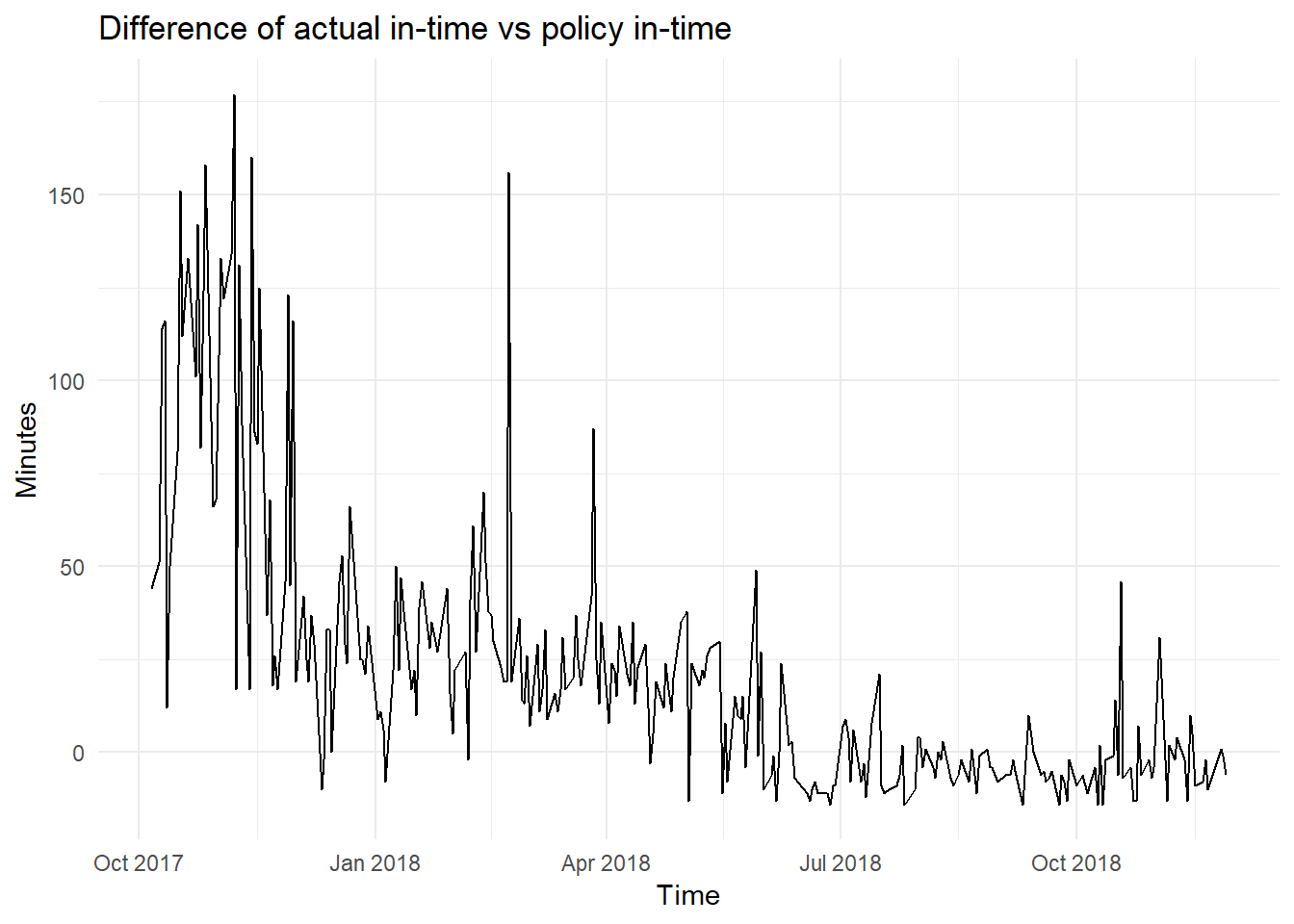A data frame can be converted to time series by using ts() if the time is continuous with no breaks. In this problem, as the data is recorded only for 5 days a week(minus holidays/leaves), I am using xts.

The autoplot() command automatically produces an appropriate plot of whatever I pass to it in the first argument. In this case, it recognizes time.series as a time series (xts) and produces a time plot.

The time plot reveals some interesting features.
1. There is a clear decreasing trend which becomes constant after July 2018.
2. There seems to be a sudden drop of difference of in-time at the end of December.
3. There seems to be an anomaly of in-time for a day during the start of March and a few other days.

## Seasonal Plots

A seasonal plot is similar to a time plot except that the data are plotted against the individual “seasons” in which the data were observed. In this problem, instead of seasons, I want to see the changes at a weekly level. The changes for 10 random weeks are:

# Adding the level at which I want to look at the plot, in this case, week level.
attendance$week.no <- paste0(year(attendance$Attendance.Date), week(attendance$Attendance.Date)) # Choosing 10 random weeks sample.week <- sample(attendance$week.no, 10)

# Plotting
ggplot(attendance %>% filter(week.no %in% sample.week),
aes(x=wday(Attendance.Date), y = diff.in.time, colour = week.no)) +
geom_line() +
scale_x_continuous(breaks=2:6, labels=c('Monday', 'Tuesday', 'Wednesday', 'Thursday', 'Friday')) +
theme_minimal() +  theme(legend.position="bottom") +
labs(x='Weekday', y='In time difference (min)', color = "Week no (YYYYDD)", title = "Weekly plots")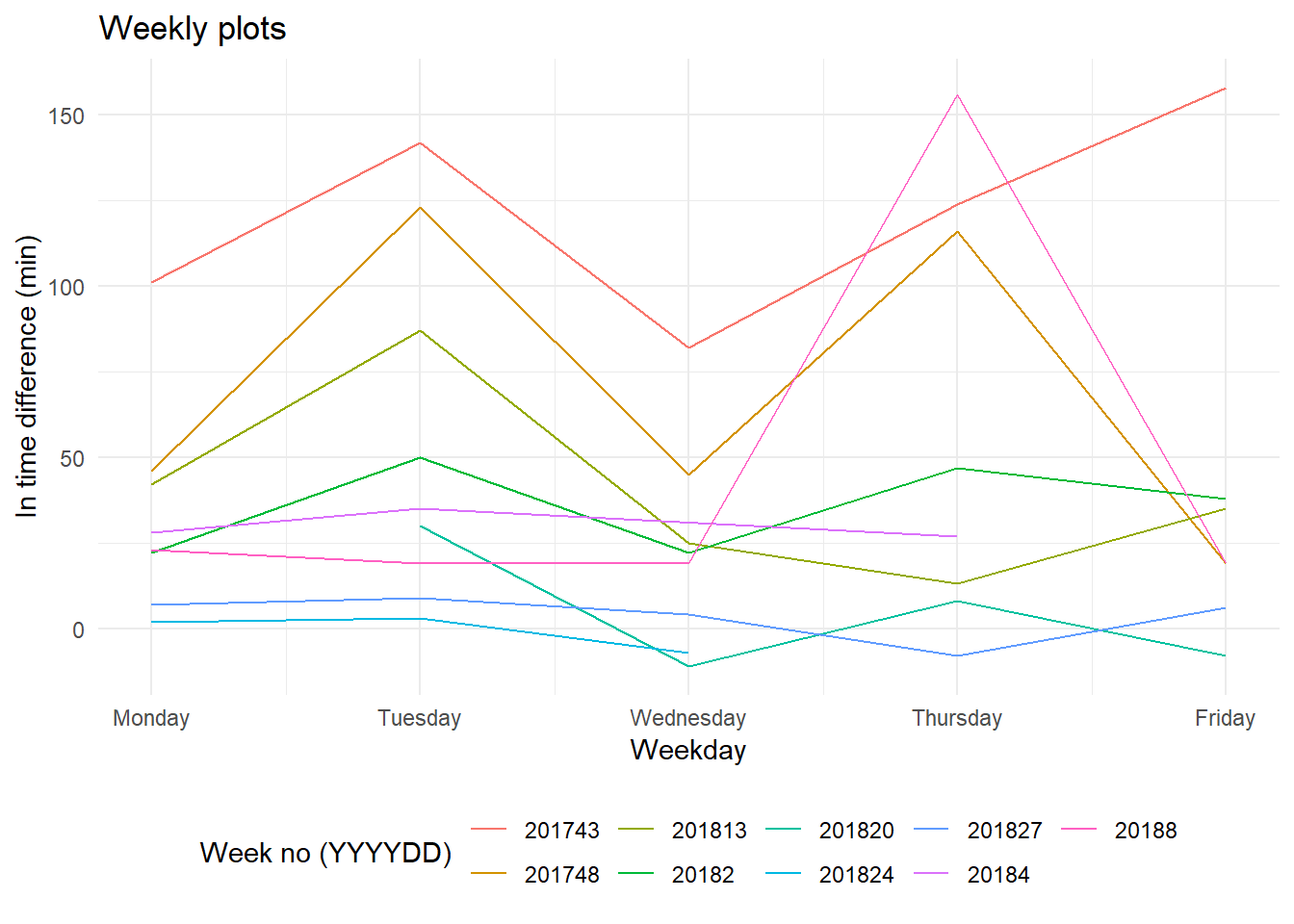This plot is exactly like the previous plot(time plot), but now the data from each week are overlapped. This plot allows the underlying weekly pattern to be seen more clearly.
1. Some values for certain weeks are missing(holidays/leaves etc). Missing value treatment should be done.
2. In-time fluctuates alternately. If I went to the office later on Monday, I was earlier on Tuesday, and so on..

A useful variation on the seasonal plot uses polar coordinates. Setting polar=TRUE makes the time series axis circular rather than horizontal, as shown below.

ggplot(data = attendance %>% filter(week.no %in% sample.week),
aes(x = wday(Attendance.Date), y = diff.in.time, colour = week.no)) +
ylim(0, NA) +
geom_polygon(fill=NA) +
coord_polar(start = 0) +
scale_x_continuous(breaks=2:6, labels=c('Mon', 'Tue', 'Wed', 'Thu', 'Fri')) +
theme_minimal() +  theme(legend.position="right") +
labs(x='', y='In time difference (min)', color = "Week no (YYYYDD)", title = "Weekly plots")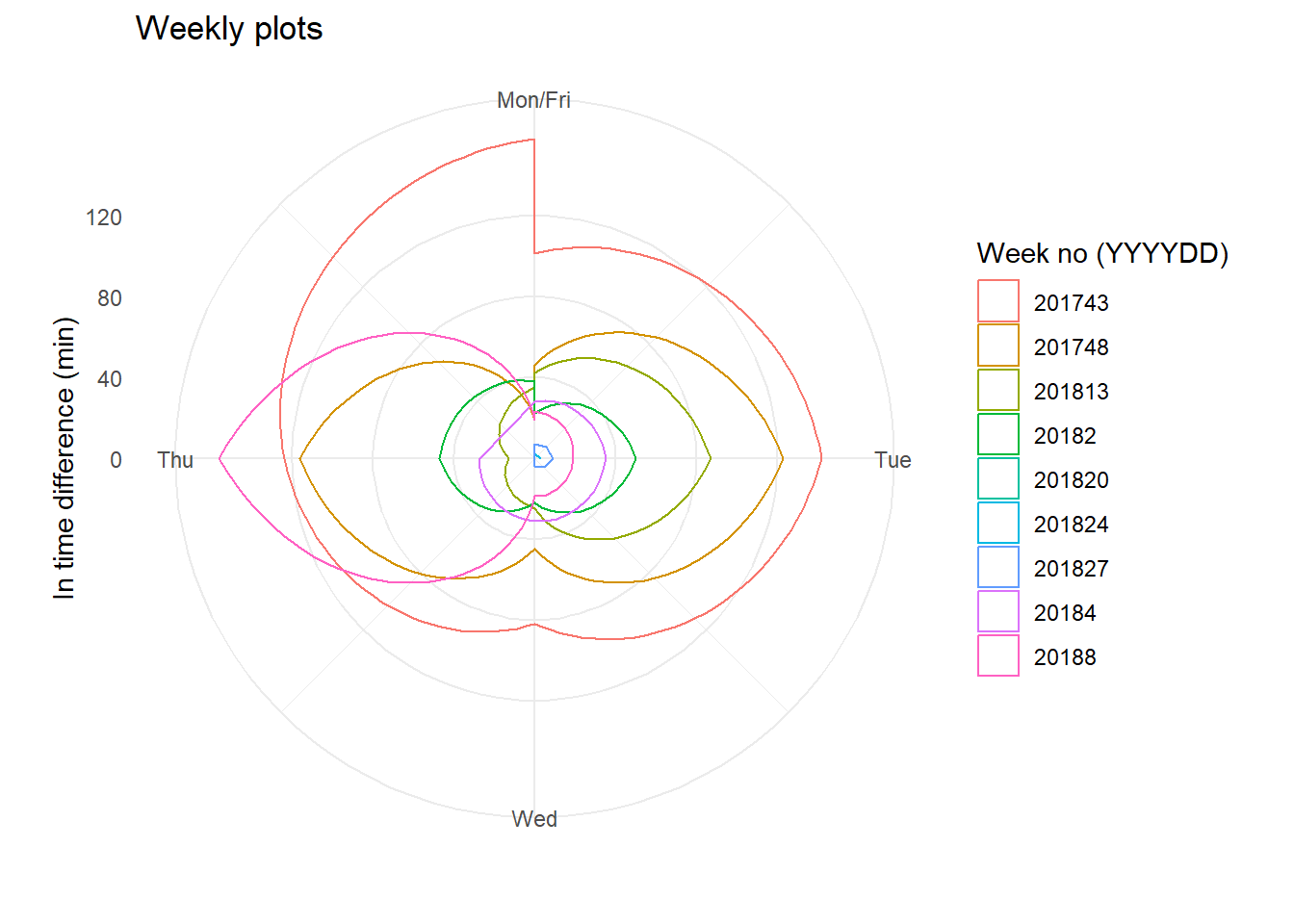## Correlogram

Just as correlation measures the extent of a linear relationship between two variables, auto-correlation measures the linear relationship between lagged values of a time series.

For example, $r_{1}$ measures the relationship between $y_{t}$ and $y_{t-1}$, $r_{2}$ measures the relationship between $y_{t}$ and $y_{t-2}$, and so on.

The value of $r_{k}$ can be written as
$r_{k} = \frac{\sum\limits_{t=k+1}^T (y_{t}-\bar{y})(y_{t-k}-\bar{y})} {\sum\limits_{t=1}^T (y_{t}-\bar{y})^2},$ where $T$ is the length of the time series.

The first 10 auto-correlation coefficients for diff.in.time are given in the following table.

 lag 1 2 3 4 5 6 7 8 9 10 corr.coeff 0.647578 0.666436 0.668575 0.568049 0.63902 0.612432 0.601126 0.615083 0.570037 0.56333

Correlogram is a plot where auto-correlation coefficients are plotted across lag to show the autocorrelation function or ACF.

ggAcf(time.series, lag.max = 50) +
theme_minimal() + ggtitle("Correlogram")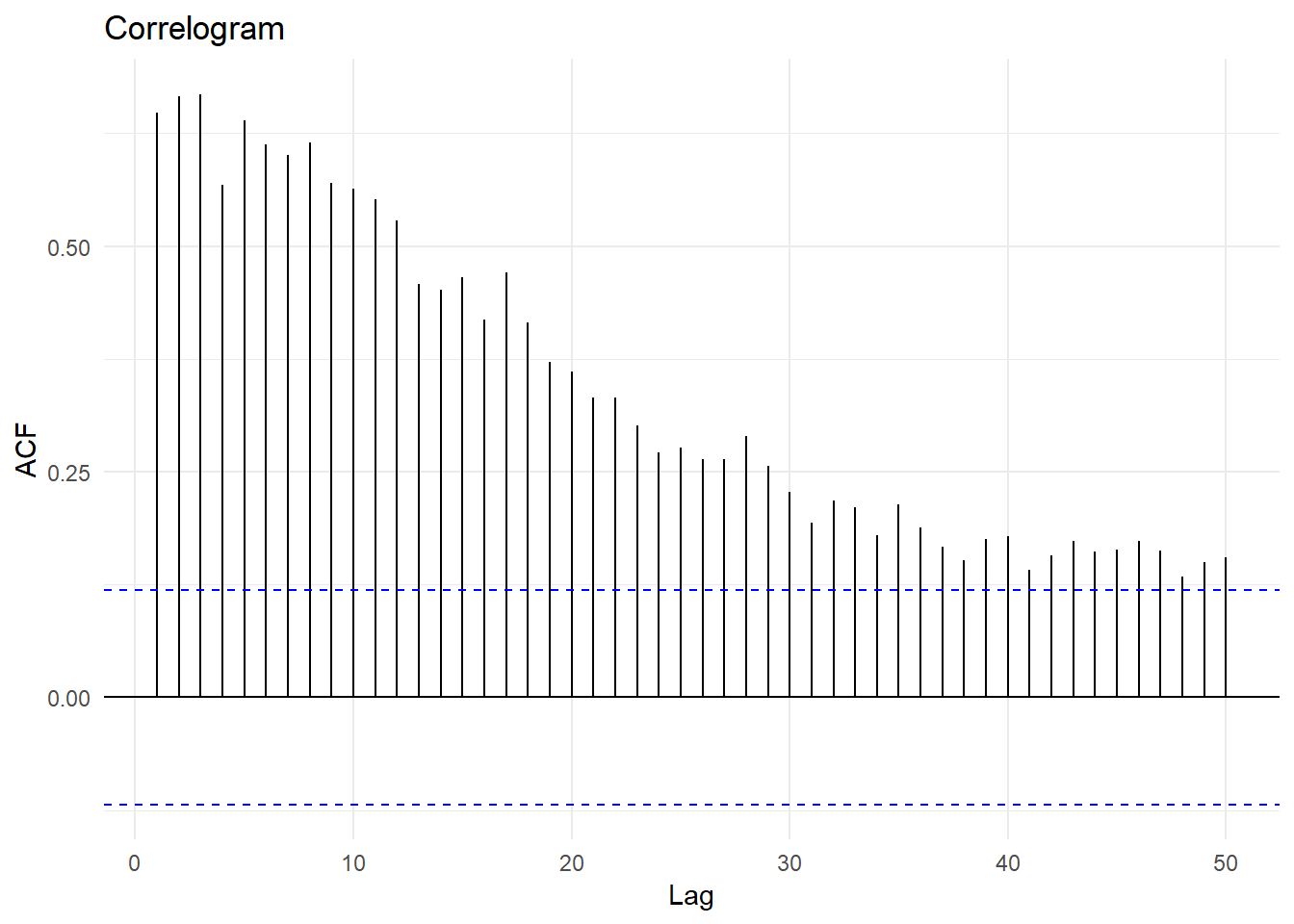When data has a trend, the auto-correlations for small lags tend to be large and positive because observations nearby in time are also nearby in size. So the ACF of a trended time series tends to have positive values that slowly decrease as the lags increase. In the above plot, I can clearly see a decreasing trend.

When data are seasonal, the auto-correlations will be larger for the seasonal lags than for other lags.

Time series that show no autocorrelation are called white noise. For white noise series, we expect each autocorrelation to be close to zero. For a white noise series, we expect 95% of the spikes in the ACF to lie within the blue dashed lines in the plot. If one or more large spikes are outside these bounds, or if substantially more than 5% of spikes are outside these bounds, then the series is probably not white noise.

When data are both trended and seasonal, you see a combination of these effects. The above plot for complete data is: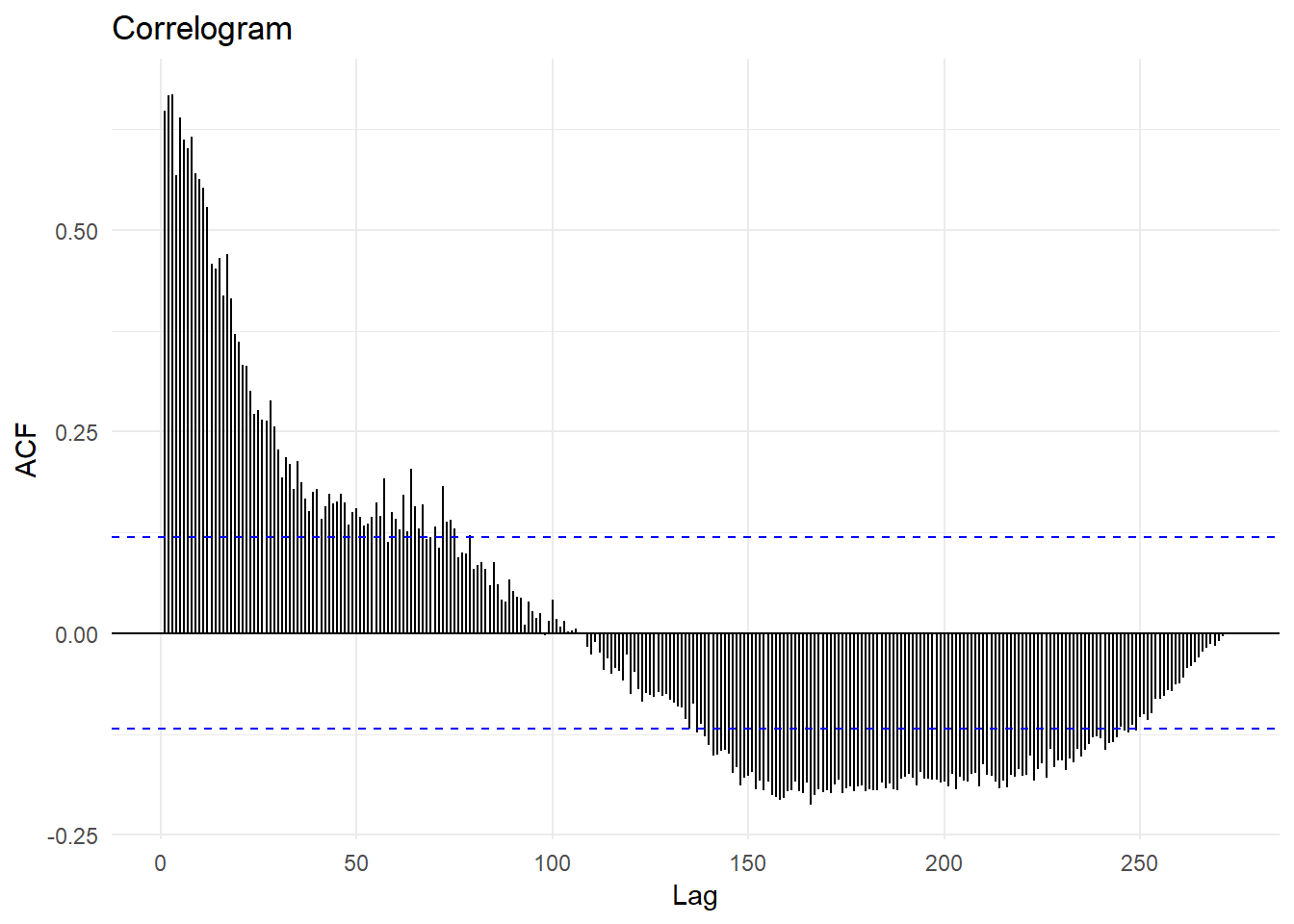## Lag plots

Lag plots are scatter-plots, where the horizontal axis shows the lagged values of the time series. Each graph shows $y_{t}$ plotted against $y_{t-k}$ for different values of $k$.

gglagplot(time.series, lags = 4, set.lags = c(1,50, 100, 150), do.lines = FALSE) +
theme_minimal()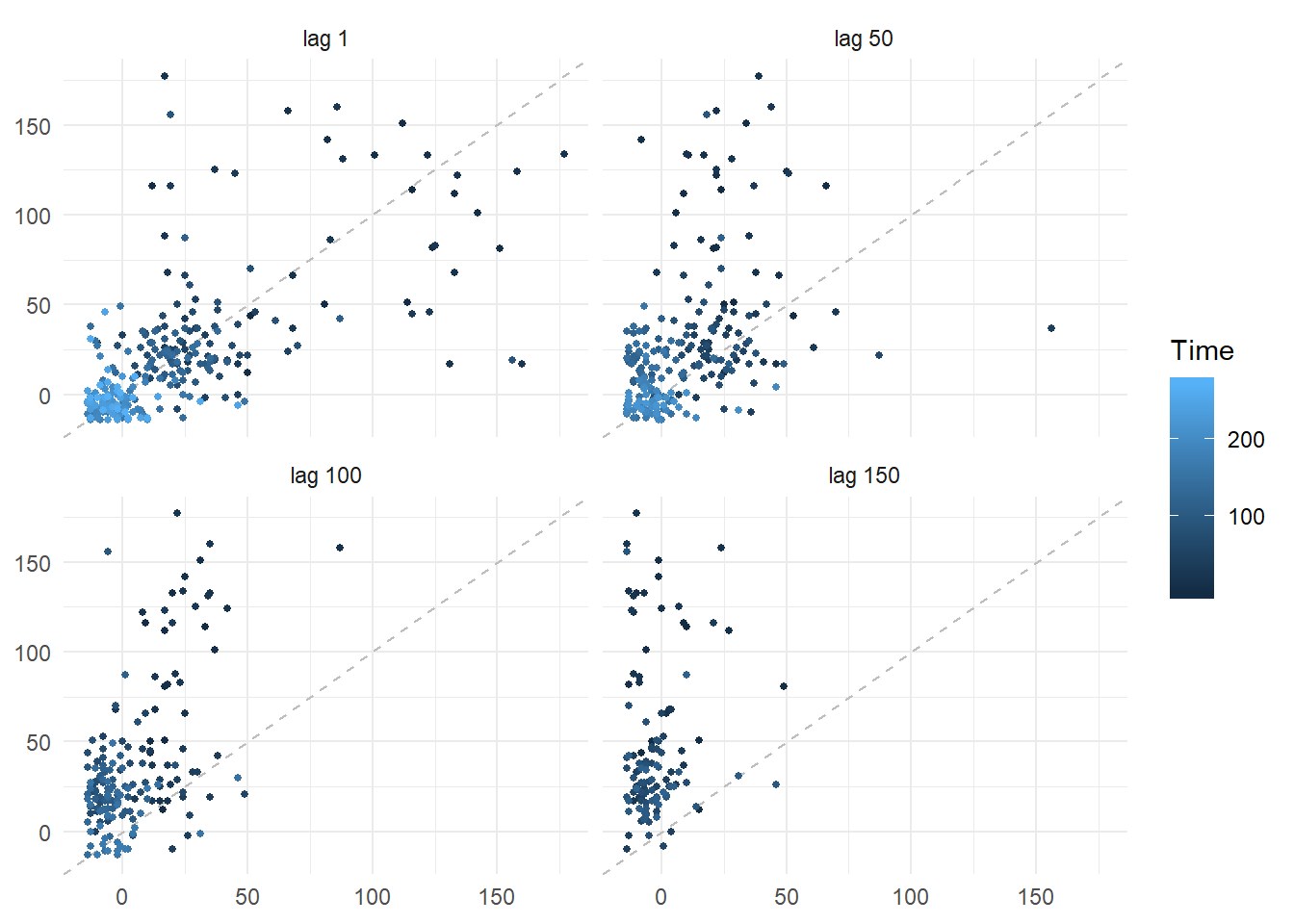If the points lie in close to the grey dotted line, then the relationship is strong at that lag. The relationship is strongly positive at lag 1, reflecting the strong seasonality in the data. For lag 50 a weak relationship while for 100, no relationship is found.

|

## 2 Replies to “Time series EDA”

1.Dheeraj says:

Nice!